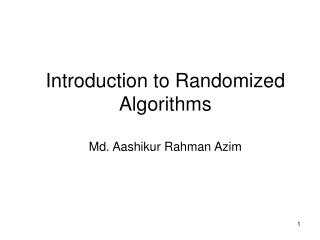Download PresentationIntroduction to Randomized Algorithms Md. Aashikur Rahman Azim

# Introduction to Randomized Algorithms Md. Aashikur Rahman Azim - PowerPoint PPT Presentation

Introduction to Randomized Algorithms Md. Aashikur Rahman Azim. Deterministic Algorithms. Goal: Prove for all input instances the algorithm solves the problem correctly and the number of steps is bounded by a polynomial in the size of the input. ALGORITHM. INPUT. OUTPUT.I am the owner, or an agent authorized to act on behalf of the owner, of the copyrighted work described.
Download Presentation## Introduction to Randomized Algorithms Md. Aashikur Rahman Azim

An Image/Link below is provided (as is) to download presentation

Download Policy: Content on the Website is provided to you AS IS for your information and personal use and may not be sold / licensed / shared on other websites without getting consent from its author.While downloading, if for some reason you are not able to download a presentation, the publisher may have deleted the file from their server.

- - - - - - - - - - - - - - - - - - - - - - - - - - E N D - - - - - - - - - - - - - - - - - - - - - - - - - -
Presentation Transcript
1. Deterministic Algorithms Goal:Prove for all input instances the algorithm solves the problem correctly and the number of steps is bounded by a polynomial in the size of the input. ALGORITHM INPUT OUTPUT

2. Randomized Algorithms • In addition to input, algorithm takes a source of random numbers and makes random choices during execution; • Behavior can vary even on a fixed input; ALGORITHM INPUT OUTPUT RANDOM NUMBERS

3. Quick Sort Select: pick an arbitrary element x in S to be the pivot. Partition: rearrange elements so that elements with value less than x go to List L to the left of x and elements with value greater than x go to the List R to the right of x. Recursion: recursively sort the lists L and R.

4. Worst Case Partitioning of Quick Sort T(n) = T(n-1) + T(0) + Theta (n) = T(n-1) + Theta(n) Proof it mathematically.

5. Best Case Partitioning of Quick Sort T(n) <= 2T(n/2) + Theta(n) Proof it mathematically.

6. Average Case of Quick Sort T(n) <= T(9n/10) + T(n/10) + cn

7. Randomized Quick Sort Randomized-Partition(A, p, r) 1. i  Random(p, r) 2. exchange A[r]  A[i] 3. returnPartition(A, p, r) Randomized-Quicksort(A, p, r) 1. ifp < r 2. thenq Randomized-Partition(A, p, r) 3. Randomized-Quicksort(A, p , q-1) 4. Randomized-Quicksort(A, q+1, r)

8. Randomized Quick Sort • Exchange A[r] with an element chosen at random from A[p…r] in Partition. • The pivot element is equally likely to be any of input elements. • For any given input, the behavior of Randomized Quick Sort is determined not only by the input but also by the random choices of the pivot. • We add randomization to Quick Sort to obtain for any input the expected performance of the algorithm to be good.

9. Analysis of Randomized Quick Sort

10. Linearity of Expectation If X1, X2, …, Xn are random variables, then

11. z2 z3 z7 z9 z8 z5 z4 z1 z6 z10 Notation • Rename the elements of A as z1, z2, . . . , zn, with zi being the ith smallest element (Rank “i”). • Define the set Zij = {zi , zi+1, . . . , zj } be the set of elements between zi and zj, inclusive. 2 9 8 3 5 4 1 6 10 7

12. Expected Number of Total Comparisons in PARTITION indicator random variable Let Xij = I {zi is compared to zj } Let X be the total number of comparisons performed by the algorithm. Then The expected number of comparisons performed by the algorithm is by linearity of expectation

13. z2 z3 z7 z9 z8 z5 z4 z1 z6 z10 2 9 8 3 5 4 1 6 10 7 Z1,6= {1, 2, 3, 4, 5, 6} {7} Z8,9 = {8, 9, 10} Comparisons in PARTITION Observation 1: Each pair of elements is compared at most once during the entire execution of the algorithm • Elements are compared only to the pivot point! • Pivot point is excluded from future calls to PARTITION Observation 2: Only the pivot is compared with elements in both partitions pivot Elements between different partitions are never compared

14. Comparisons in PARTITION z2 z3 z7 z9 z8 z5 z4 z1 z6 z10 2 9 8 3 5 4 1 6 10 7 Case 1: pivot chosen such as: zi < x < zj • ziand zj will never be compared Case 2: zi or zj is the pivot • zi and zjwill be compared • only if one of them is chosen as pivot before any other element in range zito zj Z1,6= {1, 2, 3, 4, 5, 6} {7} Z8,9 = {8, 9, 10}

15. Expected Number of Comparisons in PARTITION Pr {Zi is compared with Zj} = Pr{Zi or Zj is chosen as pivot before other elements in Zi,j} = 2 / (j-i+1) = O(nlgn)